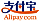Bookcover of Statistical Aspects of Growth Models
Booktitle:

# Statistical Aspects of Growth Models

## Growth Model Methodology

LAP LAMBERT Academic Publishing (2013-03-24 )eligible for voucher
ISBN-13:

### 978-3-659-36889-9

ISBN-10:
365936889X
EAN:
9783659368899
Book language:
English
Blurb/Shorttext:
Growth Modelling is the heart of various fields of Applied Statistics such as Bio-metrics, Econometrics,Demometrics,Business and Industrial Statistics. This book brought out the mathematical and Statistical aspects of growth and developed some new statistical growth models by using the logistic and Poisson regressions, Inferential aspects of these statistical growth models have also been described in this book.Firstly, a Logistic regression model has been specified as a number of family of generalized linear models.Secondly, a Poisson regression model has been specified by using the Poisson probability model for count data.Thirdly, residuals are obtained from Logistic and Poisson regression models, to test for the adequacy of the models; Fourthly, a test procedure has been developed for assessing the fit of the Multiple Logistic regression Fifthly, new Multiple Logistic regression growth and Multiple Poisson regression growth models have been specified by using logistic and Poisson regressions. Finally, a criterion for choosing between Logistic regression growth and Poisson regression growth models has been discussed by testing the nonlinear hypotheses.
Publishing house:
Website:
https://www.lap-publishing.com/
By (author) :
S.Asif Alisha, Balasiddamuni Pagadala Stella Ingilela
Number of pages:
276
Published at:
2013-03-24
Stock:
Available
Category:
Theory of probability, stochastics, mathematical statistics
Price:
74.90 €
Keywords:
Statistics### Categories